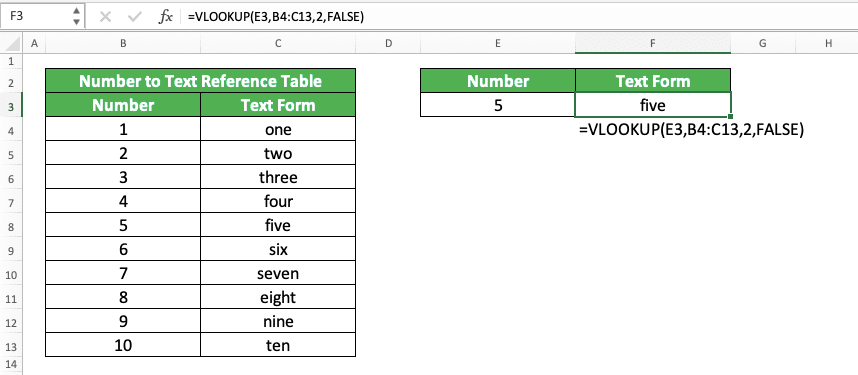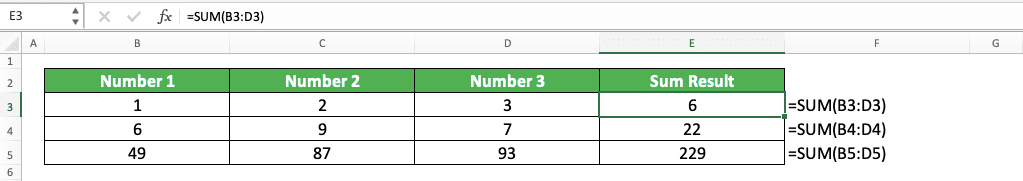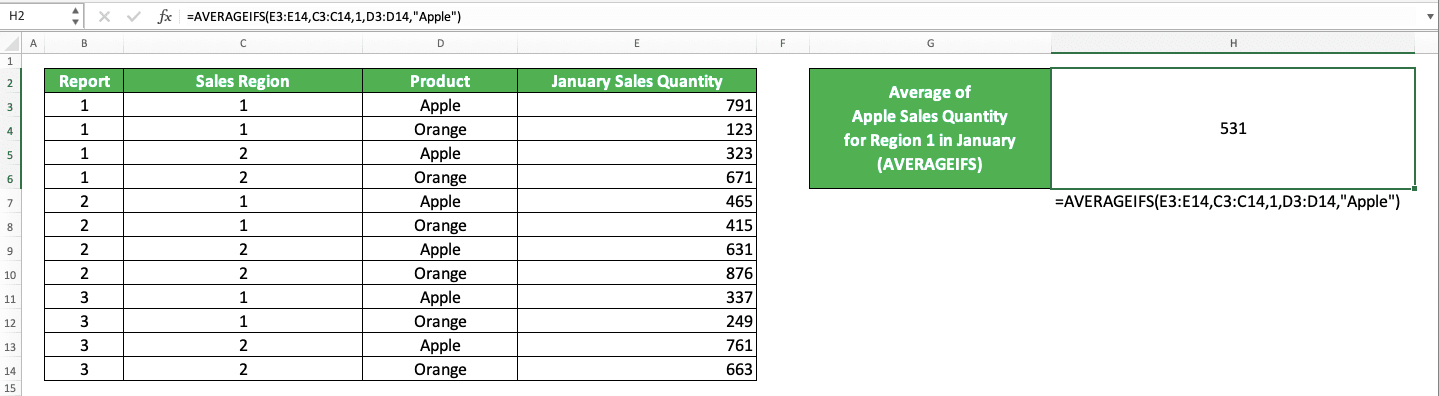8 Basic Excel Formulas and How to Use Them + Examples - Compute Expert

# 8 Basic Excel Formulas and How to Use Them + Examples

Home >> Excel Tutorials from Compute Expert >> Excel Tips and Trick >> 8 Basic Excel Formulas and How to Use Them + Examples

In this tutorial, you will learn about the eight basic excel formulas and their brief usage explanation plus examples.

If you have used excel, then you might have already known that formula is an important feature in the software. It has many variations, with each has a unique function that can help us to process our data.

However, these many variations also make it hard for us to master them all. Therefore, it will be great if we know the basic excel formulas we should understand instead. This is so, at least, we can do most of our general work optimally in excel using them.

We create this tutorial to help you with that. Hopefully, after you learn here, you can do your daily excel work, which needs formulas to help, with much less problem!

Disclaimer: This post may contain affiliate links from which we earn commission from qualifying purchases/actions at no additional cost for you. Learn more

## 8 Basic Excel Formulas and How to Use Them + Examples

Among many formulas we can use in excel, according to experience, there are 8 of them we most often use. Mastering their usage should help you to do most of your work in excel which requires formulas to complete.

### IFThis formula is probably the formula excel users most often use. As we often need logic processing for our work in excel, this IF formula can help you to do just that.

IF helps you to produce data depending on the value of the logic condition you input in it. If the logic condition value is true, then IF will give you its true result. Otherwise, if the logic condition value is false, then IF will give you its false result.

Generally, the writing form of IF in excel is like this.

= IF ( logic_condition , result_if_true , result_if_false )

Whenever you need to get a result according to some logic, use IF to help you!

### VLOOKUPWhen you need a formula to look up for data, the first one that comes to your mind is probably VLOOKUP. As we often have reference tables in excel, we need VLOOKUP to get the data we need from them.

VLOOKUP looks up your data by searching for its reference value input in the cell range you specify. From there, it gets that value’s row position to get your data in the column you want in the cell range.

You can write VLOOKUP in excel like this.

= VLOOKUP ( reference_value , cell_range , result_col_index , search_mode )

There is also a much more flexible alternative to VLOOKUP in excel, which is INDEX MATCH (or XLOOKUP if you have a newer excel version). If you have the time, then you should learn how to use INDEX MATCH or XLOOKUP. Once you master them, you might not want to use VLOOKUP again to find your data!

### SUMOne of the calculation processes we often use to our numbers is the sum process. The SUM formula helps you to do this calculation fast in excel as we often put our numbers in cell ranges.

Just input all the numbers you want to sum to your SUM formula and you are good to go. SUM will give you the result you need immediately!

Here is how you write SUM generally in excel.

= SUM ( number1 , number2 , … )

You can input the numbers you want to sum in the form of direct numbers, cells, or cell ranges. Don’t forget to give commas ( , ) if you give more than one inputs to your SUM.

### SUMIFSSUM is good if you want to sum numbers just normally. However, you may sometimes have criteria for the data entries which numbers you want to sum. For this kind of sum process, you need SUMIFS.

The way to use SUMIFS in excel isn’t too hard. Just input the cell range containing the numbers you want to sum first. After that, input the cell ranges containing the data you want to evaluate with your criteria and the criteria themselves. The cell ranges you input should be parallel and have the same size.

The general writing form of SUMIFS is like this.

= SUMIFS ( number_range , data_range1 , criterion1 , … )

The data ranges and the criteria in SUMIFS are in pairs. Input them as many as the criteria you have for your sum process.

### COUNTAAnother data processing process you may often do in excel is counting the amount of data you have. We may need it to check the completeness of our data or get the input we need for further data processing.

If you just need to count the amount of data you have, then COUNTA can help you with that. Just input the cell ranges where you want to count your data to COUNTA and you will get your counting result!

Here is its general writing form in excel.

= COUNTA ( value1 , value2 , … )

We can input direct data, cells, or cell ranges to COUNTA. However, we usually input only one cell range to this formula.

Whenever you need the information of your data amount in excel, don’t hesitate to use COUNTA!

### COUNTIFSWhat if we have some criteria for the data entries we want to count the amount of? There is also a formula for that, which is COUNTIFS.

Just input the columns/rows of the cell ranges you want to count the amount of data in. After that, input the criteria to evaluate the data in those columns/rows. As in SUMIFS, you must input the cell ranges and the criteria in pairs.

Generally, here is the writing form of COUNTIFS in excel.

= COUNTIFS ( data_range1 , criterion1 , … )

You should input parallel and same size cell ranges (usually in the form of columns/rows) to COUNTIFS so the counting logic process becomes easier.

### AVERAGEBesides sum, another calculation process we often need to do on our numbers is average. To average your numbers, excel provides AVERAGE to help you do it fast.

The writing of AVERAGE in excel is quite similar to SUM as you can see below.

= AVERAGE ( number1 , number2 , … )

You can input direct numbers, cells, and cell ranges in AVERAGE. However, we usually just input one cell range which contains all the numbers we want to average.

### AVERAGEIFSYou may also sometimes have criteria for the data entries which numbers you want to average. AVERAGEIFS can help you to process the calculation with that condition in mind.

Here is the general writing form of AVERAGEIFS in excel.

= AVERAGEIFS ( number_range , data_range1 , criterion1 , … )

The inputs of AVERAGEIFS are similar to SUMIFS. You need to provide the numbers’ cell range first before you input the data cell ranges and their criteria pairs.

Criteria-based calculations are something we often do in excel. AVERAGEIFS can do it for you when it comes to averaging your numbers with criteria.

***

So, what do you think about those formulas? Have you mastered them all so you can work in excel more optimally?

Of course, there are other formulas in excel which can help you with your data processing process too. It is certainly better if you can master more excel formulas as you can use them when the condition is appropriate.

However, mastering the eight formulas we mentioned above should give you a strong base to do basic works in excel. They should be enough if you process data that is general and not too complex.

Want to do some exercises to deepen your understanding about the eight formulas we mention here? Click on their Compute Expert tutorial links to find the exercises and start doing them!

Other tutorials you should learn:

Get updated excel info from Compute Expert by registering your email. It's free!